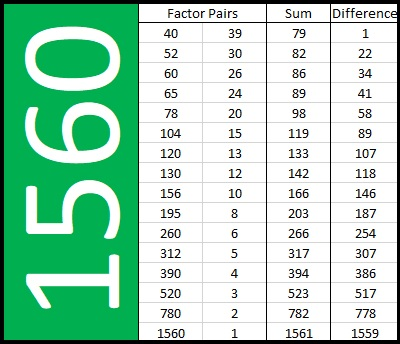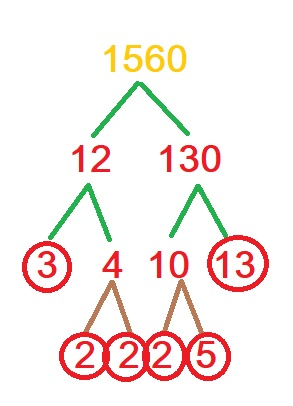# Why Do Factor Pairs of 1560 Make Sum-Difference?

Contents

### Today’s Puzzle:

1560 has 16 different factor pairs. One of those pairs sum up to 89, and another pair subtracts to 89. It is only the 50th time that the sum of a factor pair of a number equals the difference of one of its other factor pairs.

You may have seen other Sum-Difference Puzzles where I’ve paired one puzzle with another puzzle and mentioned that the second puzzle was really the first puzzle in disguise. That is not the case for this puzzle because 89 is a prime number. This puzzle is a primitive; there is not a simpler puzzle that is its equivalent. Don’t let that worry you, however, everything you need to solve this puzzle can be found in this post.Although I am making a big deal about our number 1560, it is the 89 which allows this puzzle to exist in the first place. You see, 89 is the hypotenuse of a Pythagorean triple, (39-80-89), and thus, 39² + 80² = 89². Since that triple is a primitive, the sum-difference is a primitive as well.

Note that (40)(39) = 1560.
We want to use the quadratic formula to solve 40x² + 89x + 39 = 0 and 40x² + 89x – 39 = 0. Let’s combine the left sides of those two equations into one expression:
40x² + 89x ± 39.
The discriminant would be 89² – 4(40)(±39)
= 89² ± 4(40)(39)
= 89² ± 2(80)(39)
= 39² + 80² ± 2(80)(39), ( That’s because 39² + 80² = 89².)
= 80² ± 2(80)(39) + 39²
= (80 ± 39)², a perfect square!

That perfect square makes 1560 one of those relatively rare numbers with factor pairs that make sum-difference.

### Factors of 1560:

• 1560 is a composite number.
• Prime factorization: 1560 = 2 × 2 × 2 × 3 × 5 × 13 , which can be written 1560 = 2³ × 3 × 5 × 13.
• 1560 has at least one exponent greater than 1 in its prime factorization so √1560 can be simplified. Taking the factor pair from the factor pair table below with the largest square number factor, we get √1560 = (√4)(√390) = 2√390.
• The exponents in the prime factorization are 2, 1, and 2. Adding one to each exponent and multiplying we get (3 + 1)(1 + 1)(1 + 1) (1 + 1) = 4 × 2 × 2 × 2 = 32. Therefore 1560 has exactly 32 factors.
• The factors of 1560 are outlined with their factor pair partners in the graphic below.That’s a lot of factor pairs for one number! Here’s a graphic showing those same factor pairs but with their sums and differences included:Find the 89 in the Sum column and the 89 in the Difference column, and you will see the factor pairs by those 89’s that make the sum and difference needed to solve the puzzle.

### More about the Number 1560:

1560 = 39 × 40, so 1560 is the sum of the first 39 even numbers:
2 + 4 + 6 + 8 + . . . + 74 + 76 + 78 = 1560.

1560 is the hypotenuse of FOUR Pythagorean triples:
384-1512-1560, which is 24 times (16-63-65),
600-1440-1560, which is (5-12-13) times 120,
792-1344-1560, which is 24 times (33-56-65), and
936-1248-1560, which is (3-4-5) times 312.

Since 1560 is a hypotenuse four different ways, could it be the bottom part of four different Sum-Difference puzzles? Yes!

You can find the top part of the puzzle by finding one half of the product of the first two numbers in each triple:
384 × 1512 ÷ 2 = ____________,
600 × 1440 ÷ 2 = ____________,
792 × 1344 ÷ 2 = ____________,
936 × 1248 ÷ 2 = ____________.

The numbers that go in the blanks are all between 100 thousand and one million, but each one of those numbers will have a factor pair that adds up to 1560 as well as another one that subtracts to 1560. If you find them, go ahead and brag about it! It will be quite an accomplishment!

### A Factor Tree for 1560:

Here is one of MANY possible factor trees for 1560:This site uses Akismet to reduce spam. Learn how your comment data is processed.